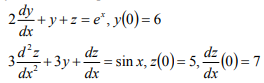Courses

# Differential Equation

## 16 Questions MCQ Test | Differential Equation

Description
This mock test of Differential Equation for Engineering Mathematics helps you for every Engineering Mathematics entrance exam. This contains 16 Multiple Choice Questions for Engineering Mathematics Differential Equation (mcq) to study with solutions a complete question bank. The solved questions answers in this Differential Equation quiz give you a good mix of easy questions and tough questions. Engineering Mathematics students definitely take this Differential Equation exercise for a better result in the exam. You can find other Differential Equation extra questions, long questions & short questions for Engineering Mathematics on EduRev as well by searching above.
QUESTION: 1

### The order of the differential equation of all tangent lines to the parabola y = x2 is

Solution:

Sol.[A]   The parametric form of the given equation is x = t, y = t2. The equation of any tangent at
t is 2xt = y t2. Differentiating, we get 2t = y1. Putting this value in the equation of tangent, we have 2 x y1/2 = y (y1/2)2 Þ 4xy1 = 4y y12

The order of this equation is one.

QUESTION: 2

Solution:
QUESTION: 3

### The general solution of (x2 D2 – xD), y= 0 is :

Solution:
QUESTION: 4

which is the linear differential equations

Solution:
QUESTION: 5

The maximum number of linearly independent solution of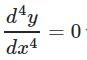with y(0)=1 is

Solution:
QUESTION: 6

If x = 2 ln cot t and y = tan t + cot t, the value of dy / dx is

Solution: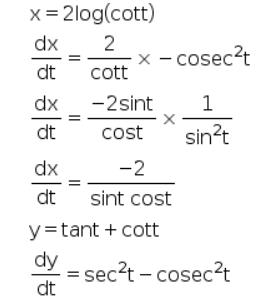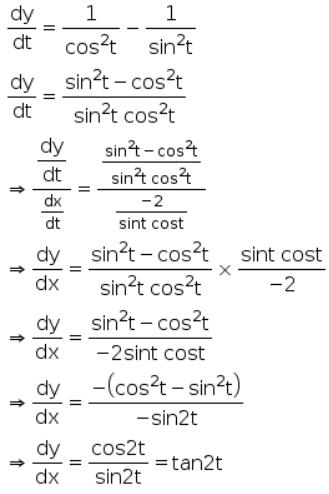QUESTION: 7

Solution of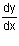+ 2xy = y is

Solution:
QUESTION: 8

The general solution of (x2 D2 – xD), y= 0 is :

Solution:
QUESTION: 9

The differential equation formed by y= acosx + bsinx + 4 where a and b are arbitrary constants is :

Solution:

y = acosx + bsinx + 4

⟹dy/dx ​= −asinx + bcosx

⟹d2y​/dx= −acosx − bsinx

⟹d2y​/dx= −y + 4

⟹ d2y​/dx+ y = 4

QUESTION: 10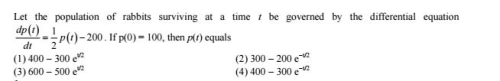Solution: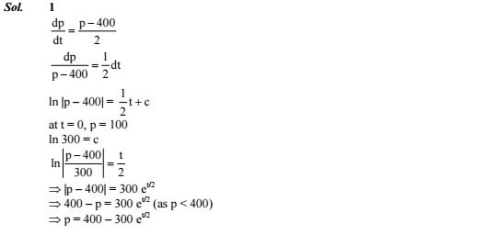QUESTION: 11

The polynomial ar2+br+c=0 is called

Solution:
QUESTION: 12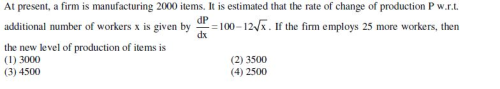Solution: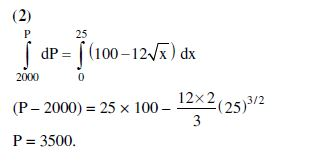QUESTION: 13

The general solution of (x2 D2 – xD), y= 0 is :

Solution:
QUESTION: 14

Linear second order ordinary differential equation is non homogeneous if

Solution:
QUESTION: 15

The polynomial ar2+br+c=0 is called

Solution:
QUESTION: 16

A differential equation is considered to be ordinary if it has

Solution: